# Forward Collision Warning Using Sensor Fusion

This example shows how to perform forward collision warning by fusing data from vision and radar sensors to track objects in front of the vehicle.

### Overview

Forward collision warning (FCW) is an important feature in driver assistance and automated driving systems, where the goal is to provide correct, timely, and reliable warnings to the driver before an impending collision with the vehicle in front. To achieve the goal, vehicles are equipped with forward-facing vision and radar sensors. Sensor fusion is required to increase the probability of accurate warnings and minimize the probability of false warnings.

For the purposes of this example, a test car (the ego vehicle) was equipped with various sensors and their outputs were recorded. The sensors used for this example were:

1. Vision sensor, which provided lists of observed objects with their classification and information about lane boundaries. The object lists were reported 10 times per second. Lane boundaries were reported 20 times per second.

2. Radar sensor with medium and long range modes, which provided lists of unclassified observed objects. The object lists were reported 20 times per second.

3. IMU, which reported the speed and turn rate of the ego vehicle 20 times per second.

4. Video camera, which recorded a video clip of the scene in front of the car. Note: This video is not used by the tracker and only serves to display the tracking results on video for verification.

The process of providing a forward collision warning comprises the following steps:

1. Obtain the data from the sensors.

2. Fuse the sensor data to get a list of tracks, i.e., estimated positions and velocities of the objects in front of the car.

3. Issue warnings based on the tracks and FCW criteria. The FCW criteria are based on the Euro NCAP AEB test procedure and take into account the relative distance and relative speed to the object in front of the car.

The visualization in this example is done using monoCamera and birdsEyePlot. For brevity, the functions that create and update the display were moved to helper functions outside of this example. For more information on how to use these displays, see Annotate Video Using Detections in Vehicle Coordinates and Visualize Sensor Coverage, Detections, and Tracks.

This example is a script, with the main body shown here and helper routines in the form of local functions in the sections that follow. For more details about local functions, see Add Functions to Scripts.

% Set up the display [videoReader, videoDisplayHandle, bepPlotters, sensor] = helperCreateFCWDemoDisplay('01_city_c2s_fcw_10s.mp4', 'SensorConfigurationData.mat'); % Read the recorded detections file [visionObjects, radarObjects, inertialMeasurementUnit, laneReports, ... timeStep, numSteps] = readSensorRecordingsFile('01_city_c2s_fcw_10s_sensor.mat'); % An initial ego lane is calculated. If the recorded lane information is % invalid, define the lane boundaries as straight lines half a lane % distance on each side of the car laneWidth = 3.6; % meters egoLane = struct('left', [0 0 laneWidth/2], 'right', [0 0 -laneWidth/2]); % Prepare some time variables time = 0; % Time since the beginning of the recording currentStep = 0; % Current timestep snapTime = 9.3; % The time to capture a snapshot of the display % Initialize the tracker [tracker, positionSelector, velocitySelector] = setupTracker(); while currentStep < numSteps && ishghandle(videoDisplayHandle) % Update scenario counters currentStep = currentStep + 1; time = time + timeStep; % Process the sensor detections as objectDetection inputs to the tracker [detections, laneBoundaries, egoLane] = processDetections(... visionObjects(currentStep), radarObjects(currentStep), ... inertialMeasurementUnit(currentStep), laneReports(currentStep), ... egoLane, time); % Using the list of objectDetections, return the tracks, updated to time confirmedTracks = updateTracks(tracker, detections, time); % Find the most important object and calculate the forward collision % warning mostImportantObject = findMostImportantObject(confirmedTracks, egoLane, positionSelector, velocitySelector); % Update video and birds-eye plot displays frame = readFrame(videoReader); % Read video frame helperUpdateFCWDemoDisplay(frame, videoDisplayHandle, bepPlotters, ... laneBoundaries, sensor, confirmedTracks, mostImportantObject, positionSelector, ... velocitySelector, visionObjects(currentStep), radarObjects(currentStep)); % Capture a snapshot if time >= snapTime && time < snapTime + timeStep snapnow; end end 

### Create the Multi-Object Tracker

The multiObjectTracker tracks the objects around the ego vehicle based on the object lists reported by the vision and radar sensors. By fusing information from both sensors, the probability of a false collision warning is reduced.

The setupTracker function returns the multiObjectTracker. When creating a multiObjectTracker, consider the following:

1. FilterInitializationFcn: The likely motion and measurement models. In this case, the objects are expected to have a constant acceleration motion. Although you can configure a linear Kalman filter for this model, initConstantAccelerationFilter configures an extended Kalman filter. See the 'Define a Kalman filter' section.

2. AssignmentThreshold: How far detections can fall from tracks. The default value for this parameter is 30. If there are detections that are not assigned to tracks, but should be, increase this value. If there are detections that get assigned to tracks that are too far, decrease this value. This example uses 35.

3. DeletionThreshold: When a track is confirmed, it should not be deleted on the first update that no detection is assigned to it. Instead, it should be coasted (predicted) until it is clear that the track is not getting any sensor information to update it. The logic is that if the track is missed P out of Q times it should be deleted. The default value for this parameter is 5-out-of-5. In this case, the tracker is called 20 times a second and there are two sensors, so there is no need to modify the default.

4. ConfirmationThreshold: The parameters for confirming a track. A new track is initialized with every unassigned detection. Some of these detections might be false, so all the tracks are initialized as 'Tentative'. To confirm a track, it has to be detected at least M times in N tracker updates. The choice of M and N depends on the visibility of the objects. This example uses the default of 2 detections out of 3 updates.

The outputs of setupTracker are:

• tracker - The multiObjectTracker that is configured for this case.

• positionSelector - A matrix that specifies which elements of the State vector are the position: position = positionSelector * State

• velocitySelector - A matrix that specifies which elements of the State vector are the velocity: velocity = velocitySelector * State

 function [tracker, positionSelector, velocitySelector] = setupTracker() tracker = multiObjectTracker(... 'FilterInitializationFcn', @initConstantAccelerationFilter, ... 'AssignmentThreshold', 35, 'ConfirmationThreshold', [2 3], ... 'DeletionThreshold', 5); % The State vector is: % In constant velocity: State = [x;vx;y;vy] % In constant acceleration: State = [x;vx;ax;y;vy;ay] % Define which part of the State is the position. For example: % In constant velocity: [x;y] = [1 0 0 0; 0 0 1 0] * State % In constant acceleration: [x;y] = [1 0 0 0 0 0; 0 0 0 1 0 0] * State positionSelector = [1 0 0 0 0 0; 0 0 0 1 0 0]; % Define which part of the State is the velocity. For example: % In constant velocity: [x;y] = [0 1 0 0; 0 0 0 1] * State % In constant acceleration: [x;y] = [0 1 0 0 0 0; 0 0 0 0 1 0] * State velocitySelector = [0 1 0 0 0 0; 0 0 0 0 1 0]; end 

### Define a Kalman Filter

The multiObjectTracker defined in the previous section uses the filter initialization function defined in this section to create a Kalman filter (linear, extended, or unscented). This filter is then used for tracking each object around the ego vehicle.

function filter = initConstantAccelerationFilter(detection) % This function shows how to configure a constant acceleration filter. The % input is an objectDetection and the output is a tracking filter. % For clarity, this function shows how to configure a trackingKF, % trackingEKF, or trackingUKF for constant acceleration. % % Steps for creating a filter: % 1. Define the motion model and state % 2. Define the process noise % 3. Define the measurement model % 4. Initialize the state vector based on the measurement % 5. Initialize the state covariance based on the measurement noise % 6. Create the correct filter % Step 1: Define the motion model and state % This example uses a constant acceleration model, so: STF = @constacc; % State-transition function, for EKF and UKF STFJ = @constaccjac; % State-transition function Jacobian, only for EKF % The motion model implies that the state is [x;vx;ax;y;vy;ay] % You can also use constvel and constveljac to set up a constant % velocity model, constturn and constturnjac to set up a constant turn % rate model, or write your own models. % Step 2: Define the process noise dt = 0.05; % Known timestep size sigma = 1; % Magnitude of the unknown acceleration change rate % The process noise along one dimension Q1d = [dt^4/4, dt^3/2, dt^2/2; dt^3/2, dt^2, dt; dt^2/2, dt, 1] * sigma^2; Q = blkdiag(Q1d, Q1d); % 2-D process noise % Step 3: Define the measurement model MF = @fcwmeas; % Measurement function, for EKF and UKF MJF = @fcwmeasjac; % Measurement Jacobian function, only for EKF % Step 4: Initialize a state vector based on the measurement % The sensors measure [x;vx;y;vy] and the constant acceleration model's % state is [x;vx;ax;y;vy;ay], so the third and sixth elements of the % state vector are initialized to zero. state = [detection.Measurement(1); detection.Measurement(2); 0; detection.Measurement(3); detection.Measurement(4); 0]; % Step 5: Initialize the state covariance based on the measurement % noise. The parts of the state that are not directly measured are % assigned a large measurement noise value to account for that. L = 100; % A large number relative to the measurement noise stateCov = blkdiag(detection.MeasurementNoise(1:2,1:2), L, detection.MeasurementNoise(3:4,3:4), L); % Step 6: Create the correct filter. % Use 'KF' for trackingKF, 'EKF' for trackingEKF, or 'UKF' for trackingUKF FilterType = 'EKF'; % Creating the filter: switch FilterType case 'EKF' filter = trackingEKF(STF, MF, state,... 'StateCovariance', stateCov, ... 'MeasurementNoise', detection.MeasurementNoise(1:4,1:4), ... 'StateTransitionJacobianFcn', STFJ, ... 'MeasurementJacobianFcn', MJF, ... 'ProcessNoise', Q ... ); case 'UKF' filter = trackingUKF(STF, MF, state, ... 'StateCovariance', stateCov, ... 'MeasurementNoise', detection.MeasurementNoise(1:4,1:4), ... 'Alpha', 1e-1, ... 'ProcessNoise', Q ... ); case 'KF' % The ConstantAcceleration model is linear and KF can be used % Define the measurement model: measurement = H * state % In this case: % measurement = [x;vx;y;vy] = H * [x;vx;ax;y;vy;ay] % So, H = [1 0 0 0 0 0; 0 1 0 0 0 0; 0 0 0 1 0 0; 0 0 0 0 1 0] % % Note that ProcessNoise is automatically calculated by the % ConstantAcceleration motion model H = [1 0 0 0 0 0; 0 1 0 0 0 0; 0 0 0 1 0 0; 0 0 0 0 1 0]; filter = trackingKF('MotionModel', '2D Constant Acceleration', ... 'MeasurementModel', H, 'State', state, ... 'MeasurementNoise', detection.MeasurementNoise(1:4,1:4), ... 'StateCovariance', stateCov); end end 

### Process and Format the Detections

The recorded information must be processed and formatted before it can be used by the tracker. This has the following steps:

1. Filtering out unnecessary radar clutter detections. The radar reports many objects that correspond to fixed objects, which include: guard-rails, the road median, traffic signs, etc. If these detections are used in the tracking, they create false tracks of fixed objects at the edges of the road and therefore must be removed before calling the tracker. Radar objects are considered nonclutter if they are either stationary in front of the car or moving in its vicinity.

2. Formatting the detections as input to the tracker, i.e., an array of objectDetection elements. See the processVideo and processRadar supporting functions at the end of this example.

 function [detections,laneBoundaries, egoLane] = processDetections... (visionFrame, radarFrame, IMUFrame, laneFrame, egoLane, time) % Inputs: % visionFrame - objects reported by the vision sensor for this time frame % radarFrame - objects reported by the radar sensor for this time frame % IMUFrame - inertial measurement unit data for this time frame % laneFrame - lane reports for this time frame % egoLane - the estimated ego lane % time - the time corresponding to the time frame % Remove clutter radar objects [laneBoundaries, egoLane] = processLanes(laneFrame, egoLane); realRadarObjects = findNonClutterRadarObjects(radarFrame.object,... radarFrame.numObjects, IMUFrame.velocity, laneBoundaries); % Return an empty list if no objects are reported % Counting the total number of objects detections = {}; if (visionFrame.numObjects + numel(realRadarObjects)) == 0 return; end % Process the remaining radar objects detections = processRadar(detections, realRadarObjects, time); % Process video objects detections = processVideo(detections, visionFrame, time); end 

### Update the Tracker

To update the tracker, call the updateTracks method with the following inputs:

1. tracker - The multiObjectTracker that was configured earlier. See the 'Create the Multi-Object Tracker' section.

2. detections - A list of objectDetection objects that was created by processDetections

3. time - The current scenario time.

The output from the tracker is a struct array of tracks.

### Find the Most Important Object and Issue a Forward Collision Warning

The most important object (MIO) is defined as the track that is in the ego lane and is closest in front of the car, i.e., with the smallest positive x value. To lower the probability of false alarms, only confirmed tracks are considered.

Once the MIO is found, the relative speed between the car and MIO is calculated. The relative distance and relative speed determine the forward collision warning. There are 3 cases of FCW:

1. Safe (green): There is no car in the ego lane (no MIO), the MIO is moving away from the car, or the distance to the MIO remains constant.

2. Caution (yellow): The MIO is moving closer to the car, but is still at a distance above the FCW distance. FCW distance is calculated using the Euro NCAP AEB Test Protocol. Note that this distance varies with the relative speed between the MIO and the car, and is greater when the closing speed is higher.

3. Warn (red): The MIO is moving closer to the car, and its distance is less than the FCW distance,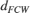.

Euro NCAP AEB Test Protocol defines the following distance calculation: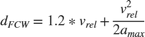where:is the forward collision warning distance.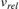is the relative velocity between the two vehicles.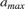is the maximum deceleration, defined to be 40% of the gravity acceleration.

 function mostImportantObject = findMostImportantObject(confirmedTracks,egoLane,positionSelector,velocitySelector) % Initialize outputs and parameters MIO = []; % By default, there is no MIO trackID = []; % By default, there is no trackID associated with an MIO FCW = 3; % By default, if there is no MIO, then FCW is 'safe' threatColor = 'green'; % By default, the threat color is green maxX = 1000; % Far enough forward so that no track is expected to exceed this distance gAccel = 9.8; % Constant gravity acceleration, in m/s^2 maxDeceleration = 0.4 * gAccel; % Euro NCAP AEB definition delayTime = 1.2; % Delay time for a driver before starting to brake, in seconds positions = getTrackPositions(confirmedTracks, positionSelector); velocities = getTrackVelocities(confirmedTracks, velocitySelector); for i = 1:numel(confirmedTracks) x = positions(i,1); y = positions(i,2); relSpeed = velocities(i,1); % The relative speed between the cars, along the lane if x < maxX && x > 0 % No point checking otherwise yleftLane = polyval(egoLane.left, x); yrightLane = polyval(egoLane.right, x); if (yrightLane <= y) && (y <= yleftLane) maxX = x; trackID = i; MIO = confirmedTracks(i).TrackID; if relSpeed < 0 % Relative speed indicates object is getting closer % Calculate expected braking distance according to % Euro NCAP AEB Test Protocol d = abs(relSpeed) * delayTime + relSpeed^2 / 2 / maxDeceleration; if x <= d % 'warn' FCW = 1; threatColor = 'red'; else % 'caution' FCW = 2; threatColor = 'yellow'; end end end end end mostImportantObject = struct('ObjectID', MIO, 'TrackIndex', trackID, 'Warning', FCW, 'ThreatColor', threatColor); end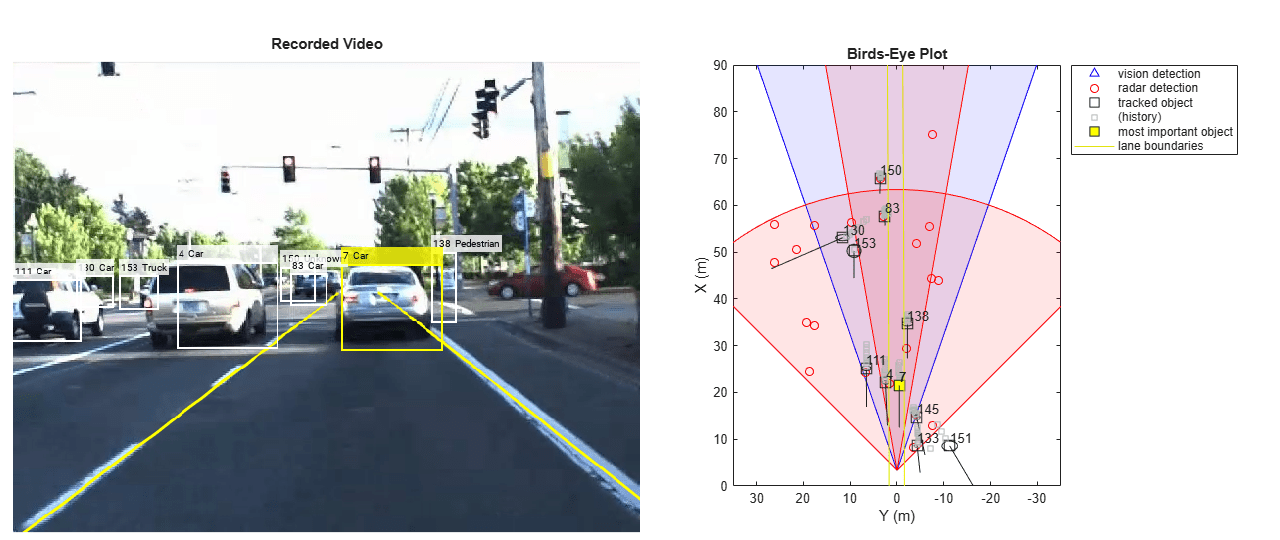### Summary

This example showed how to create a forward collision warning system for a vehicle equipped with vision, radar, and IMU sensors. It used objectDetection objects to pass the sensor reports to the multiObjectTracker object that fused them and tracked objects in front of the ego vehicle.

Try using different parameters for the tracker to see how they affect the tracking quality. Try modifying the tracking filter to use trackingKF or trackingUKF, or to define a different motion model, e.g., constant velocity or constant turn. Finally, you can try to define your own motion model.

### Supporting Functions

function [visionObjects, radarObjects, inertialMeasurementUnit, laneReports, ... timeStep, numSteps] = readSensorRecordingsFile(sensorRecordingFileName) % Read Sensor Recordings % The |ReadDetectionsFile| function reads the recorded sensor data file. % The recorded data is a single structure that is divided into the % following substructures: % % # |inertialMeasurementUnit|, a struct array with fields: timeStamp, % velocity, and yawRate. Each element of the array corresponds to a % different timestep. % # |laneReports|, a struct array with fields: left and right. Each element % of the array corresponds to a different timestep. % Both left and right are structures with fields: isValid, confidence, % boundaryType, offset, headingAngle, and curvature. % # |radarObjects|, a struct array with fields: timeStamp (see below), % numObjects (integer) and object (struct). Each element of the array % corresponds to a different timestep. % |object| is a struct array, where each element is a separate object, % with the fields: id, status, position(x;y;z), velocity(vx,vy,vz), % amplitude, and rangeMode. % Note: z is always constant and vz=0. % # |visionObjects|, a struct array with fields: timeStamp (see below), % numObjects (integer) and object (struct). Each element of the array % corresponds to a different timestep. % |object| is a struct array, where each element is a separate object, % with the fields: id, classification, position (x;y;z), % velocity(vx;vy;vz), size(dx;dy;dz). Note: z=vy=vz=dx=dz=0 % % The timeStamp for recorded vision and radar objects is a uint64 variable % holding microseconds since the Unix epoch. Timestamps are recorded about % 50 milliseconds apart. There is a complete synchronization between the % recordings of vision and radar detections, therefore the timestamps are % not used in further calculations. A = load(sensorRecordingFileName); visionObjects = A.vision; radarObjects = A.radar; laneReports = A.lane; inertialMeasurementUnit = A.inertialMeasurementUnit; timeStep = 0.05; % Data is provided every 50 milliseconds numSteps = numel(visionObjects); % Number of recorded timesteps end 

processLanes Converts sensor-reported lanes to parabolicLaneBoundary lanes and maintains a persistent ego lane estimate

function [laneBoundaries, egoLane] = processLanes(laneReports, egoLane) % Lane boundaries are updated based on the laneReports from the recordings. % Since some laneReports contain invalid (isValid = false) reports or % impossible parameter values (-1e9), these lane reports are ignored and % the previous lane boundary is used. leftLane = laneReports.left; rightLane = laneReports.right; % Check the validity of the reported left lane cond = (leftLane.isValid && leftLane.confidence) && ... ~(leftLane.headingAngle == -1e9 || leftLane.curvature == -1e9); if cond egoLane.left = cast([leftLane.curvature, leftLane.headingAngle, leftLane.offset], 'double'); end % Update the left lane boundary parameters or use the previous ones leftParams = egoLane.left; leftBoundaries = parabolicLaneBoundary(leftParams); leftBoundaries.Strength = 1; % Check the validity of the reported right lane cond = (rightLane.isValid && rightLane.confidence) && ... ~(rightLane.headingAngle == -1e9 || rightLane.curvature == -1e9); if cond egoLane.right = cast([rightLane.curvature, rightLane.headingAngle, rightLane.offset], 'double'); end % Update the right lane boundary parameters or use the previous ones rightParams = egoLane.right; rightBoundaries = parabolicLaneBoundary(rightParams); rightBoundaries.Strength = 1; laneBoundaries = [leftBoundaries, rightBoundaries]; end 

function realRadarObjects = findNonClutterRadarObjects(radarObject, numRadarObjects, egoSpeed, laneBoundaries) % The radar objects include many objects that belong to the clutter. % Clutter is defined as a stationary object that is not in front of the % car. The following types of objects pass as nonclutter: % % # Any object in front of the car % # Any moving object in the area of interest around the car, including % objects that move at a lateral speed around the car % Allocate memory normVs = zeros(numRadarObjects, 1); inLane = zeros(numRadarObjects, 1); inZone = zeros(numRadarObjects, 1); % Parameters LaneWidth = 3.6; % What is considered in front of the car ZoneWidth = 1.7*LaneWidth; % A wider area of interest minV = 1; % Any object that moves slower than minV is considered stationary for j = 1:numRadarObjects [vx, vy] = calculateGroundSpeed(radarObject(j).velocity(1),radarObject(j).velocity(2),egoSpeed); normVs(j) = norm([vx,vy]); laneBoundariesAtObject = computeBoundaryModel(laneBoundaries, radarObject(j).position(1)); laneCenter = mean(laneBoundariesAtObject); inLane(j) = (abs(radarObject(j).position(2) - laneCenter) <= LaneWidth/2); inZone(j) = (abs(radarObject(j).position(2) - laneCenter) <= max(abs(vy)*2, ZoneWidth)); end realRadarObjectsIdx = union(... intersect(find(normVs > minV), find(inZone == 1)), ... find(inLane == 1)); realRadarObjects = radarObject(realRadarObjectsIdx); end 

calculateGroundSpeed Calculates the true ground speed of a radar-reported object from the relative speed and the ego vehicle speed

function [Vx,Vy] = calculateGroundSpeed(Vxi,Vyi,egoSpeed) % Inputs % (Vxi,Vyi) : relative object speed % egoSpeed : ego vehicle speed % Outputs % [Vx,Vy] : ground object speed Vx = Vxi + egoSpeed; % Calculate longitudinal ground speed theta = atan2(Vyi,Vxi); % Calculate heading angle Vy = Vx * tan(theta); % Calculate lateral ground speed end 

processVideo Converts reported vision objects to a list of objectDetection objects

function postProcessedDetections = processVideo(postProcessedDetections, visionFrame, t) % Process the video objects into objectDetection objects numRadarObjects = numel(postProcessedDetections); numVisionObjects = visionFrame.numObjects; if numVisionObjects classToUse = class(visionFrame.object(1).position); visionMeasCov = cast(diag([2,2,2,100]), classToUse); % Process Vision Objects: for i=1:numVisionObjects object = visionFrame.object(i); postProcessedDetections{numRadarObjects+i} = objectDetection(t,... [object.position(1); object.velocity(1); object.position(2); 0], ... 'SensorIndex', 1, 'MeasurementNoise', visionMeasCov, ... 'MeasurementParameters', {1}, ... 'ObjectClassID', object.classification, ... 'ObjectAttributes', {object.id, object.size}); end end end 

processRadar Converts reported radar objects to a list of objectDetection objects

function postProcessedDetections = processRadar(postProcessedDetections, realRadarObjects, t) % Process the radar objects into objectDetection objects numRadarObjects = numel(realRadarObjects); if numRadarObjects classToUse = class(realRadarObjects(1).position); radarMeasCov = cast(diag([2,2,2,100]), classToUse); % Process Radar Objects: for i=1:numRadarObjects object = realRadarObjects(i); postProcessedDetections{i} = objectDetection(t, ... [object.position(1); object.velocity(1); object.position(2); object.velocity(2)], ... 'SensorIndex', 2, 'MeasurementNoise', radarMeasCov, ... 'MeasurementParameters', {2}, ... 'ObjectAttributes', {object.id, object.status, object.amplitude, object.rangeMode}); end end end 

fcwmeas The measurement function used in this forward collision warning example

function measurement = fcwmeas(state, sensorID) % The example measurements depend on the sensor type, which is reported by % the MeasurementParameters property of the objectDetection. The following % two sensorID values are used: % sensorID=1: video objects, the measurement is [x;vx;y]. % sensorID=2: radar objects, the measurement is [x;vx;y;vy]. % The state is: % Constant velocity state = [x;vx;y;vy] % Constant turn state = [x;vx;y;vy;omega] % Constant acceleration state = [x;vx;ax;y;vy;ay] if numel(state) < 6 % Constant turn or constant velocity switch sensorID case 1 % video measurement = [state(1:3); 0]; case 2 % radar measurement = state(1:4); end else % Constant acceleration switch sensorID case 1 % video measurement = [state(1:2); state(4); 0]; case 2 % radar measurement = [state(1:2); state(4:5)]; end end end 

fcwmeasjac The Jacobian of the measurement function used in this forward collision warning example

function jacobian = fcwmeasjac(state, sensorID) % The example measurements depend on the sensor type, which is reported by % the MeasurementParameters property of the objectDetection. We choose % sensorID=1 for video objects and sensorID=2 for radar objects. The % following two sensorID values are used: % sensorID=1: video objects, the measurement is [x;vx;y]. % sensorID=2: radar objects, the measurement is [x;vx;y;vy]. % The state is: % Constant velocity state = [x;vx;y;vy] % Constant turn state = [x;vx;y;vy;omega] % Constant acceleration state = [x;vx;ax;y;vy;ay] numStates = numel(state); jacobian = zeros(4, numStates, 'like', state); if numel(state) < 6 % Constant turn or constant velocity switch sensorID case 1 % video jacobian(1,1) = 1; jacobian(2,2) = 1; jacobian(3,3) = 1; case 2 % radar jacobian(1,1) = 1; jacobian(2,2) = 1; jacobian(3,3) = 1; jacobian(4,4) = 1; end else % Constant acceleration switch sensorID case 1 % video jacobian(1,1) = 1; jacobian(2,2) = 1; jacobian(3,4) = 1; case 2 % radar jacobian(1,1) = 1; jacobian(2,2) = 1; jacobian(3,4) = 1; jacobian(4,5) = 1; end end end